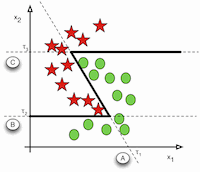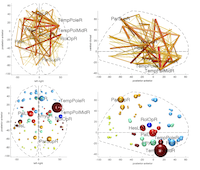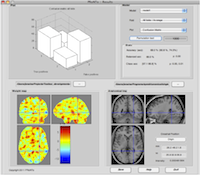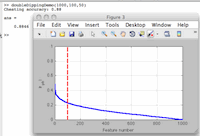Here I provide some code that is useful or instructive for pattern recognition and neuroimaging.

## Connectivity decoding toolkitWe have a partial matlab toolbox to allow you to replicate the experiments in our NeuroImage paper on connectivity-based decoding. In short, for each subject, the procedure is

1. Preprocess the data: use this Matlab preprocessing code.
2. Denoise the data (take care of motion and physiological artefacts): code release in preparation.
3. Filter into subbands: use this Matlab filtering code, or Sophie Achard's R package Brainwaver.
4. Compute dependency between time-series: use this Matlab dependency computation code, the Matlab corr function, or Sophie Achard's R package Brainwaver.
5. Embed into vector space: code release in preparation
Also download the example main script and the connectivity decoding toolkit helper (uncompress in the same directory as the above code).

After the 4th step, it is a simple procedure to perform the direct embedding proposed in our paper. Once the connectivity graphs are represented in vector space, any classical statistical machine learning technique can be used to perform decoding. Email me in case you need assistance.

## Visualising brain graphsGiven an adjacency matrix representing a graph and a codebook giving spatial coordinates and names of vertices, you can use the Flexible Brain Graph Visualizer to display your graph exactly the way you want, including scaling, custom colormaps, split colormaps for vertices and edges, and much more. It has quite a number of options.

## Pattern Recognition on Neuroimaging Toolbox (PRoNTo)If you have used SPM before, you can easily use PRoNTo to convert your group-level mass-univariate analyses into a cross-validated predictive modelling task, with support for classification and regression.

You can also use PRoNTo without having an SPM analysis done. The toolkit is user-friendly and supports GUI, batch, and script execution. It has built-in cross-validation and safeguards to avoid double-dipping.

Check out the paper and if you like what you see, download the software!

## Comparing adjacency matrices

There are a few ways to compare adjacency matrices for graphs with the fixed-cardinality vertex sequence property (in particular brain graphs). If you just have two adjacency matrices, you can get use Mantel's test of the null hypothesis of 'no association' between the two matrices. Here is a Matlab implementation of the Mantel test. You will also need this function to do 'direct embedding' (lexicographically rearrange the upper-triangular part of a matrix into a vector). If you prefer R you can use the ade4 package.

## Double dipping demoOr, why you shouldn't learn your feature selection on your entire dataset. This is a classic and fun Matlab demo showing excellent classification accuracy from randomly generated data - and a good motivation for keeping training, evaluation, and testing sets separate! Grab the code here.

## Compiling randomforest-matlab

If you use Abhishek Jaiantilal's nice implementation of Random Forest for Matlab, you may have trouble compiling it under recent Matlab versions (R2014 and up). If mex complains about not being able to cast an int to mxComplexity in the call to mxCreateNumericArray in mex_ClassificationRF_train.cpp and mex_ClassificationRF_predict.cpp, replace the last argument in the call (0) to the constant mxREAL. It seems the function's signature has changed.